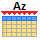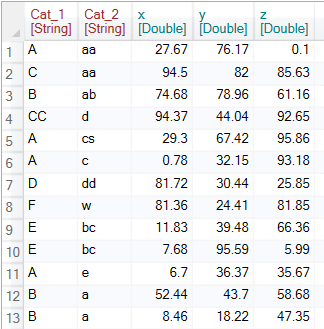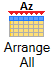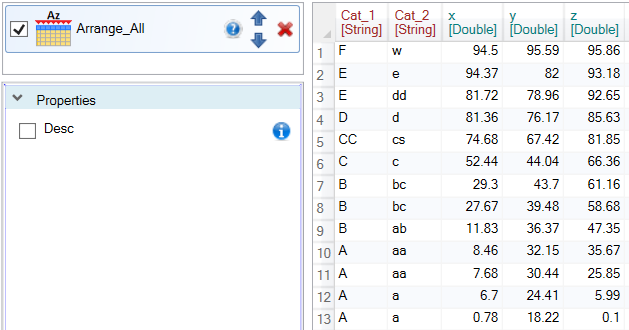#Arrange_All

Function sorts all table variables (columns) independent of each other.

## Properties

 Desc Boolean. If TRUE variables will be sorted in descending order.

## Keywords

Arrange All Columns, Arrange Variables, Sort All Columns, Sort Columns Independently, Order Rows, Order Table, Sort Table, Sort Columns At Once

## How to Sort All Excel Table Columns Independently (Individually )

Table sorting in Excel spreadsheet is a simple task. For 90%, we use the Arrange function. In some cases, when analyzing data, it is useful to display table data sorted in ascending or descending order, where this sorting process is applied to all columns at once (independently).

We break the structure of the table with this action. Basically, we obtain a list of individually sorted variables instead of the original table. Such sorting is useful if you want to get a better insight into the variability of values for individual variables (columns) or if you want to analyze extremes. An example may be chemical analysis - we can get Top N highest values for individual measured components. An example of the Arrange_All function is shown on the image below.For deeper description of the function, we use the following table, which contains two categorical (Cat_1 and Cat_2) and three numeric variables (x, y and z).If you want to sort the table columns independently from each other, click on the Arrange All button in the ribbon toolbar tab Variables.After clicking, the Arrange_All function appears in the sidebar. If you click on it, one property will show in the Properties Panel - Desc. This property determines whether variables in the table are sorted in ascending or descending order.An example in the following figure shows the setting in which all values were sorted in ascending order. If you want reverse order (descending), just set the Desc property to TRUE.Overall, this is a very simple feature that is useful in very specific cases. These cases occur, for example, if you want to analyze the extreme values of multiple variables at once - independently of each other.

If you want to sort the rows according to the selected variables (without breaking the structure of the table records - rows), use the Arrange function.